Designing noise-robust single-qubit gates for IBM Qiskit

Increasing robustness against dephasing and control noise using Q-CTRL pulses

Boulder Opal enables you to design numerically optimized controls which can improve the performance of quantum computing hardware. Specifically, robust control solutions are able to reduce sensitivity to dephasing and/or control noise, including slowly varying noise sources that arise as hardware drifts over time.

In this application note we present an entire workflow - from gate optimization through to experimental validation on IBM Quantum Hardware. We will cover:

• Designing optimized dephasing-noise-robust controls using graphs
• Incorporating band-limits on the optimization to produce smooth pulses
• Validating performance using filter functions and quasi-static-noise-susceptibility scans
• Formatting for Qiskit and experimental execution on IBM Quantum hardware.

A detailed discussion of optimized-pulse performance on IBM hardware can be found in our technical manuscript, "Error-robust quantum logic optimization using a cloud quantum computer interface", published as Phys. Rev. Applied 15, 064054 (2021).

Some cells in this notebook require an account with IBM-Q to execute correctly. If you want to run them, please go to the IBM-Q experience to set up an account.

Imports and initialization

import time
import warnings
from pathlib import Path

# Disable warning from qiskit
warnings.filterwarnings("ignore")

# Choose to run experiments or to use saved data
use_saved_data = True
use_IBM = False

if use_saved_data == False:
timestr = time.strftime("%Y%m%d-%H%M%S")
print("Time label for data saved throughout this experiment:" + timestr)
data_folder = Path(
"resources/designing-noise-robust-single-qubit-gates-for-ibm-qiskit/"
)

import jsonpickle
import matplotlib.pyplot as plt

# General imports
import numpy as np
from scipy import interpolate

from qctrlvisualizer import QCTRL_STYLE_COLORS, get_qctrl_style, plot_controls

# Q-CTRL imports
from qctrl import Qctrl

# Parameters
giga = 1.0e9
mega = 1.0e6
micro = 1.0e-6
nano = 1.0e-9
scale_factor = 1e-14

plt.style.use(get_qctrl_style())
colors = {
"Q-CTRL": QCTRL_STYLE_COLORS,
"Square": QCTRL_STYLE_COLORS,
"IBM default X-gate": QCTRL_STYLE_COLORS,
}

bloch_colors = {
"x": QCTRL_STYLE_COLORS,
"y": QCTRL_STYLE_COLORS,
"z": QCTRL_STYLE_COLORS,
}
bloch_markers = {"x": "x", "y": "s", "z": "o"}
bloch_lines = {"x": "--", "y": "-.", "z": "-"}
bloch_basis = ["x", "y", "z"]

# Q-CTRL auxiliary functions
def get_detuning_scan(scan_array, control):

durations = [segment["duration"] for segment in control["I"]]
duration = np.sum(durations)

I_values = [segment["value"] for segment in control["I"]]
Q_values = [segment["value"] for segment in control["Q"]]
shift_I = qctrl.types.quasi_static_scan.Shift(
control=[
qctrl.types.RealSegmentInput(duration=duration, value=value)
for duration, value in zip(durations, I_values)
],
operator=sigma_x / 2,
)
shift_Q = qctrl.types.quasi_static_scan.Shift(
control=[
qctrl.types.RealSegmentInput(duration=duration, value=value)
for duration, value in zip(durations, Q_values)
],
operator=sigma_y / 2,
)
drift = qctrl.types.quasi_static_scan.Drift(
operator=sigma_z,
noise=qctrl.types.quasi_static_scan.Noise(
name="dephasing noise",
values=scan_array,
),
)
target = qctrl.types.TargetInput(operator=np.array([[1, 0], [0, 0]]))

# Compute quasi-static scan
result = qctrl.functions.calculate_quasi_static_scan(
duration=duration,
shifts=[shift_Q, shift_I],
drifts=[drift],
target=target,
)
infidelities = 1 - np.array(
[sampled_point.infidelity for sampled_point in result.samples]
)

return infidelities

def get_amplitude_scan(amplitudes, control):
durations = [segment["duration"] for segment in control["I"]]
duration = np.sum(durations)
I_values = np.array([segment["value"] for segment in control["I"]])
Q_values = np.array([segment["value"] for segment in control["Q"]])
phasors = I_values + Q_values * 1j

drive = qctrl.types.quasi_static_scan.Drive(
control=[
qctrl.types.ComplexSegmentInput(duration=duration, value=value)
for duration, value in zip(durations, phasors)
],
operator=sigma_p / 2,
noise=qctrl.types.quasi_static_scan.Noise(
name="drive noise",
values=amplitudes,
),
)
target = qctrl.types.TargetInput(operator=np.array([[1, 0], [0, 0]]))

# Compute quasi-static scan
result = qctrl.functions.calculate_quasi_static_scan(
duration=duration,
drives=[drive],
target=target,
)
infidelities = 1 - np.array(
[sampled_point.infidelity for sampled_point in result.samples]
)

return infidelities

def simulation_coherent(control, time_samples):

durations = [segment["duration"] for segment in control["I"]]
duration = sum(durations)

I_values = np.array([segment["value"] for segment in control["I"]])
Q_values = np.array([segment["value"] for segment in control["Q"]])
phasors = I_values + 1j * Q_values
drive = qctrl.types.coherent_simulation.Drive(
control=[
qctrl.types.ComplexSegmentInput(duration=duration, value=value)
for duration, value in zip(durations, phasors)
],
operator=sigma_p / 2,
)

initial_state_vector = np.array([1.0, 0.0])
sample_times = np.linspace(0, duration, time_samples)
simulation = qctrl.functions.calculate_coherent_simulation(
duration=duration,
sample_times=sample_times,
drives=[drive],
initial_state_vector=initial_state_vector,
)

gate_times = np.array([sampled_point.time for sampled_point in simulation.samples])
state_vectors = np.array(
[sampled_point.state_vector for sampled_point in simulation.samples]
)

bloch_vector_components = {
"x": np.real(
np.array(
[
np.linalg.multi_dot([np.conj(state), sigma_x, state])
for state in state_vectors
]
)
),
"y": np.real(
np.array(
[
np.linalg.multi_dot([np.conj(state), sigma_y, state])
for state in state_vectors
]
)
),
"z": np.real(
np.array(
[
np.linalg.multi_dot([np.conj(state), sigma_z, state])
for state in state_vectors
]
)
),
}

return bloch_vector_components, gate_times

def save_var(file_name, var):
# Save a single variable to a file using jsonpickle
f = open(file_name, "w+")
to_write = jsonpickle.encode(var)
f.write(to_write)
f.close()

# Return a variable from a json file
f = open(file_name, "r+")
decoded = jsonpickle.decode(encoded)
f.close()
return decoded

qctrl = Qctrl()

# IBM-Q imports
if use_IBM == True:
import qiskit.pulse as pulse
from qiskit import IBMQ
from qiskit.compiler import assemble
from qiskit.pulse import (
Acquire,
AcquireChannel,
DriveChannel,
MeasureChannel,
MemorySlot,
Play,
)
from qiskit.pulse.library import Waveform
from qiskit.tools.jupyter import *
from qiskit.tools.monitor import job_monitor

# IBM credentials and backend selection
provider = IBMQ.get_provider(
)

backend = provider.get_backend("ibmq_armonk")
backend_defaults = backend.defaults()
backend_config = backend.configuration()

# Backend properties
qubit = 0
qubit_freq_est = backend_defaults.qubit_freq_est[qubit]
dt = backend_config.dt
print(f"Qubit: {qubit}")
print(f"Hardware sampling time: {dt/nano} ns")
print(f"Qubit frequency estimate: {qubit_freq_est/giga} GHz")

# Drive and measurement channels
drive_chan = DriveChannel(qubit)
meas_chan = MeasureChannel(qubit)
inst_sched_map = backend_defaults.instruction_schedule_map
measure_schedule = inst_sched_map.get(
"measure", qubits=backend_config.meas_map[qubit]
)
else:
# Use default dt for armonk backend
dt = 2 / 9 * nano
# Use 0 qubit for armonk
qubit = 0
# Use last frequency update
qubit_freq_est = 4974444325.742604
qubit_frequency_updated = qubit_freq_est
print("IBM offline parameters")
print(f"Qubit: {qubit}")
print(f"Hardware sampling time: {dt/nano} ns")
print(f"Qubit frequency estimate: {qubit_freq_est/giga} GHz")
IBM offline parameters
Qubit: 0
Hardware sampling time: 0.2222222222222222 ns
Qubit frequency estimate: 4.9744443257426045 GHz

Creation and verification of dephasing-robust Q-CTRL pulses

We use Boulder Opal to perform optimizations to achieve an X-gate operation on the qubit. The total Hamiltonian of the driven quantum system is:

$$H(t) = \left(1 + \beta_\gamma (t)\right)H_c(t) + \eta(t) \sigma_z,$$

where $\beta_\gamma(t)$ is a fractional time-dependent amplitude fluctuation process, $\eta(t)$ is a small stochastic slowly-varying dephasing noise process, and $H_c(t)$ is the control term given by

\begin{align*} H_c(t) = & \frac{1}{2}\left(\gamma^*(t) \sigma_- + \gamma(t) \sigma_+ \right) \\ = & \frac{1}{2}\left(I(t) \sigma_x + Q(t) \sigma_y \right). \end{align*}

Here, $\gamma(t) = I(t) + i Q(t)$ is the time-dependent complex-valued control pulse waveform and $\sigma_k$, $k=x,y,z$, are the Pauli matrices.

Creating dephasing-robust pulses

We begin by defining basic operators, constants, and optimization constraints. The latter are defined with the hardware backend characteristics in mind. The duration of each segment of the piecewise constant control pulse, for example, is required to be an integer multiple of the backend sampling time, dt. This guarantees that Q-CTRL pulses can be properly implemented given the time resolution of the hardware.

sigma_z = np.array([[1.0, 0.0], [0.0, -1.0]], dtype=complex)
sigma_x = np.array([[0.0, 1.0], [1.0, 0.0]], dtype=complex)
sigma_y = np.array([[0.0, -1.0j], [1.0j, 0.0]], dtype=complex)
sigma_p = np.array([[0.0, 0.0], [1.0, 0.0]], dtype=complex)
X_gate = np.array([[0.0, 1.0], [1.0, 0.0]], dtype=complex)
X90_gate = np.array([[1.0, -1j], [-1j, 1.0]], dtype=complex) / np.sqrt(2)

number_of_segments = {}
number_of_optimization_variables = {}
cutoff_frequency = {}
segment_scale = {}
duration_int = {}
duration = {}

scheme_names = ["Square", "Q-CTRL"]
rabi_rotation = np.pi
number_of_runs = 20
omega_max = 2 * np.pi * 8.5e6
I_max = omega_max / np.sqrt(2)
Q_max = omega_max / np.sqrt(2)

# Dephasing-robust pulse parameters
number_of_optimization_variables["Q-CTRL"] = 64
number_of_segments["Q-CTRL"] = 256
segment_scale["Q-CTRL"] = 5
cutoff_frequency["Q-CTRL"] = omega_max * 2
duration_int["Q-CTRL"] = number_of_segments["Q-CTRL"] * segment_scale["Q-CTRL"]
duration["Q-CTRL"] = duration_int["Q-CTRL"] * dt

In the following cell, we create a band-limited robust pulse using a sinc filter to suppress high frequencies in the pulse waveform. The optimization setup involves defining two piecewise constant signals for the $I$ and $Q$ pulses, convolving them with the sinc filter of specified cutoff frequency, and finally discretizing the output signal into the desired number of segments (256 in this case). You can find more details on how to set up optimizations using filters in this user guide.

Note that the execution cells in this notebook contain an option (set at the initialization cell) to run all the commands from scratch or to use previously saved data.

if use_saved_data == False:
robust_dephasing_controls = {}

graph = qctrl.create_graph()

# Create I & Q variables
I_values = graph.optimization_variable(
count=number_of_optimization_variables["Q-CTRL"],
lower_bound=-I_max,
upper_bound=I_max,
)
Q_values = graph.optimization_variable(
count=number_of_optimization_variables["Q-CTRL"],
lower_bound=-Q_max,
upper_bound=Q_max,
)

# Anchor ends to zero with amplitude rise/fall envelope
time_points = np.linspace(
-1.0, 1.0, number_of_optimization_variables["Q-CTRL"] + 2
)[1:-1]
envelope_function = 1.0 - np.abs(time_points)
I_values = I_values * envelope_function
Q_values = Q_values * envelope_function

# Create I & Q signals
I_signal = graph.pwc_signal(values=I_values, duration=duration["Q-CTRL"])
Q_signal = graph.pwc_signal(values=Q_values, duration=duration["Q-CTRL"])

# Apply the sinc filter
sinc_kernel = graph.sinc_convolution_kernel(cutoff_frequency["Q-CTRL"])

I_signal_filtered = graph.convolve_pwc(pwc=I_signal, kernel=sinc_kernel)
Q_signal_filtered = graph.convolve_pwc(pwc=Q_signal, kernel=sinc_kernel)

# Re-discretize signal
I_signal = graph.discretize_stf(
stf=I_signal_filtered,
duration=duration["Q-CTRL"],
segment_count=number_of_segments["Q-CTRL"],
name="I",
)
Q_signal = graph.discretize_stf(
stf=Q_signal_filtered,
duration=duration["Q-CTRL"],
segment_count=number_of_segments["Q-CTRL"],
name="Q",
)

# Create Hamiltonian control terms
I_term = I_signal * sigma_x / 2.0
Q_term = Q_signal * sigma_y / 2.0

control_hamiltonian = I_term + Q_term

# Create dephasing noise term
dephasing = graph.constant_pwc_operator(
duration=duration["Q-CTRL"], operator=sigma_z / 2.0 / duration["Q-CTRL"]
)

# Create infidelity
infidelity = graph.infidelity_pwc(
hamiltonian=control_hamiltonian,
target=graph.target(X_gate),
noise_operators=[dephasing],
name="infidelity",
)

# Calculate optimization
result = qctrl.functions.calculate_optimization(
graph=graph, cost_node_name="infidelity", output_node_names=["I", "Q"]
)

print(f"Cost: {result.cost}")

robust_dephasing_controls["Q-CTRL"] = result.output
scheme_names.append("Q-CTRL")

filename = data_folder / ("robust_dephasing_controls_" + timestr)
save_var(filename, robust_dephasing_controls)
else:
filename = data_folder / "robust_dephasing_controls_demo"

Here is a 'primitive' benchmark pulse: a square pulse with the same duration as the optimized controls to serve as a reference. This duration matches that of the default IBM-Q U3 gate in this backend.

number_of_segments["Square"] = 1
segment_scale["Square"] = 1280
duration_int["Square"] = number_of_segments["Square"] * segment_scale["Square"]
duration["Square"] = duration_int["Square"] * dt
square_pulse_durations = np.array([duration["Square"]])
square_pulse_values_control = np.array([rabi_rotation / duration["Square"]])

square_sequence = {
"I": [
{"duration": d, "value": v}
for d, v in zip(square_pulse_durations, square_pulse_values_control)
],
"Q": [
{"duration": d, "value": v}
for d, v in zip(square_pulse_durations, np.zeros())
],
}
scheme_names.append("Square")

Now, robust and primitive controls are combined into a single dictionary and plotted.

gate_schemes = {**robust_dephasing_controls, **{"Square": square_sequence}}

for scheme_name, gate in gate_schemes.items():
fig = plt.figure()
fig.suptitle(scheme_name)
plot_controls(fig, gate)
plt.show()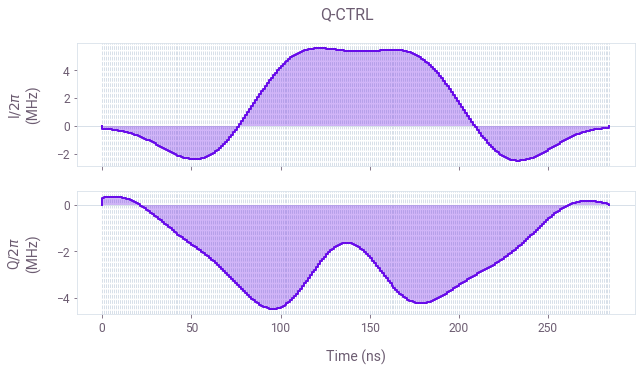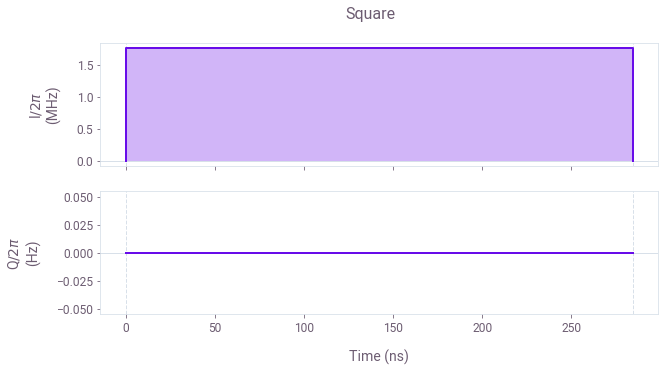The control plots represent the $I(t)$ and $Q(t)$ components of the control pulses for: Q-CTRL optimizations (top) and the primitive control (bottom). Note that the robust pulse obtained is mostly symmetrical. If desired, time-symmetrical pulses can be enforced within the optimization.

Simulated time evolution of the driven qubit

Another useful way to characterize the pulses is to use the Q-CTRL simulation tool to obtain the time evolution of the system. This way one can observe the effect of arbitrary pulses on the qubit dynamics and check if the pulses are performing the desired operation. The next two cells run the simulations, extract the results, and plot them.

# Q-CTRL simulations
simulated_bloch = {}
gate_times = {}
for scheme_name, control in gate_schemes.items():
simulated_bloch[scheme_name], gate_times[scheme_name] = simulation_coherent(
control, 100
)

# Plot results
fig, axs = plt.subplots(1, len(gate_schemes.keys()), figsize=(15, 5))
fig.suptitle(f"Time evolution under control pulses for qubit {qubit}", y=1.1)

for idx, scheme_name in enumerate(gate_schemes.keys()):
ax = axs[idx]
for meas_basis in bloch_basis:
ax.plot(
gate_times[scheme_name] / nano,
simulated_bloch[scheme_name][meas_basis],
ls=bloch_lines[meas_basis],
label=f"{meas_basis}: simulation",
)
ax.set_title(scheme_name)
ax.set_xlabel("Time (ns)")

axs.set_ylabel("Bloch components")
hs, ls = axs.get_legend_handles_labels()
fig.legend(handles=hs, labels=ls, loc="center", bbox_to_anchor=(0.5, 1.0), ncol=3)

plt.show()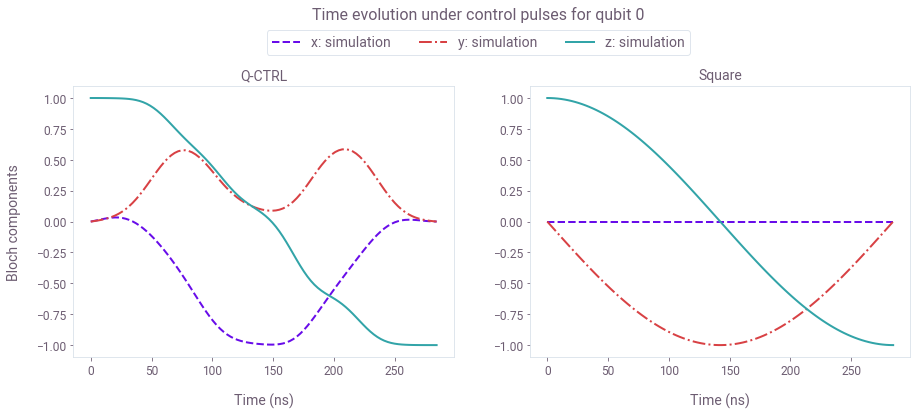The simulated Bloch vector components $(x, y, z)$ of the state dynamics for the duration of the robust and primitive pulses.

Robustness characterization with filter functions and quasi-static scan

Filter functions provide a useful way to determine the sensitivity of pulses to different time varying noise mechanisms. The next cells generate filter functions for the Q-CTRL robust pulses and the primitive square pulse under dephasing noise.

sample_count = 4096
frequencies = np.logspace(-2, np.log10(omega_max), 2000)
filter_functions = {}

for scheme_name, control in gate_schemes.items():
durations = [segment["duration"] for segment in control["I"]]
I_values = [segment["value"] for segment in control["I"]]
Q_values = [segment["value"] for segment in control["Q"]]
shift_I = qctrl.types.filter_function.Shift(
control=[
qctrl.types.RealSegmentInput(duration=duration, value=value)
for duration, value in zip(durations, I_values)
],
operator=sigma_x / 2,
)
shift_Q = qctrl.types.filter_function.Shift(
control=[
qctrl.types.RealSegmentInput(duration=duration, value=value)
for duration, value in zip(durations, Q_values)
],
operator=sigma_y / 2,
)
dephasing_noise = qctrl.types.filter_function.Drift(
noise=True,
operator=sigma_z,
)

filter_function = qctrl.functions.calculate_filter_function(
duration=np.sum(durations),
frequencies=frequencies,
sample_count=sample_count,
shifts=[shift_I, shift_Q],
drifts=[dephasing_noise],
)

filter_functions[scheme_name] = filter_function.samples
Your task calculate_filter_function (action_id="619323") has started. You can use the qctrl.get_result method to retrieve previous results.

Your task calculate_filter_function (action_id="619325") has started. You can use the qctrl.get_result method to retrieve previous results.

We next verify the pulse robustness against quasi-static noise by comparing the optimized $\pi$-pulses with the primitive pulse while detuning the drive frequency from the qubit frequency: $\Delta = \omega_\text{drive}-\omega_\text{qubit}$. This is tantamount to an effective dephasing term in the Hamiltonian, the strength and effect of which may be measured by scanning the detuning $\Delta$. The scan is calculated in the cell below.

gate_infidelity = {}
scan_array = np.linspace(-np.pi * 1.0 * mega, np.pi * 1.0 * mega, 21)
gate_infidelity["dephasing"] = {
scheme_name: get_detuning_scan(scan_array, control)
for scheme_name, control in gate_schemes.items()
}

Here's a plot of the filter functions and the detuning scan.

fig, axs = plt.subplots(1, 2, figsize=(15, 5))
fig.suptitle("Robustness verification", y=1.1)

ax = axs
ax.set_xlabel("Frequency (Hz)")
ax.set_ylabel("Inverse power")
ax.set_title("Filter functions")
ax.set_xlim(np.min(frequencies), np.max(frequencies))
for scheme_name, _data in filter_functions.items():
inverse_powers = np.array([_d.inverse_power for _d in _data])
inverse_power_precisions = np.array([_d.inverse_power_uncertainty for _d in _data])

ax.loglog(
frequencies, inverse_powers, "-", label=scheme_name, color=colors[scheme_name]
)
y_upper = inverse_powers + inverse_power_precisions
y_lower = inverse_powers - inverse_power_precisions
ax.fill_between(
frequencies,
y_lower,
y_upper,
hatch="||",
alpha=0.35,
facecolor="none",
edgecolor=colors[scheme_name],
linewidth=0.0,
)

ax = axs
ax.set_title("Robustness scan")
ax.set_ylabel("Ground state population")
ax.set_xlabel("Detuning (MHz)")
for name, infidelity in gate_infidelity["dephasing"].items():
ax.plot(scan_array * 1e-6 / np.pi, infidelity, label=name, color=colors[name])

hs, ls = axs.get_legend_handles_labels()
fig.legend(handles=hs, labels=ls, loc="center", bbox_to_anchor=(0.5, 1.0), ncol=3)

plt.show()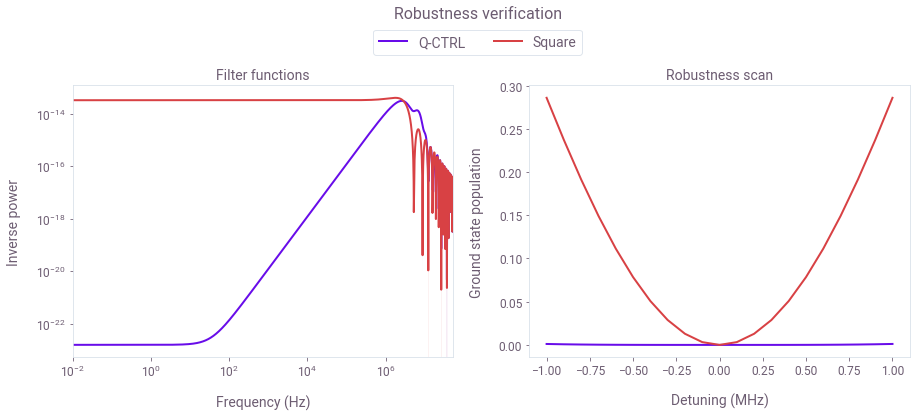Left plot: Filter functions for the control pulses under dephasing noise. The hallmark of robustness is the significant drop of the filter function values at low frequencies. This feature is clearly demonstrated for the optimized Q-CTRL pulses. Right plot: Theoretical ground state population as a function of the detuning. The Q-CTRL solution shows flat behavior for a large range of detunings as compared to the primitive pulse, indicating robustness against dephasing.

Experimental time evolution of the driven qubit

We now compare the implementation and performance of the pulses discussed previously. A good test to check that the Q-CTRL pulses are being implemented properly in the IBM hardware is to compare the theoretical with the experimental time evolution of the qubit state. The next cell executes the different steps to obtain such a comparison:

• Calibrate pulses for the specific IBM hardware,
• Set up the schedules and run them on IBM-Q,
• Extract and plot the resulting dynamics.

Note that here we use Rabi rate calibration data obtained previously for the ibmq_armonk device. To learn how to calibrate devices see the How to automate calibration of control hardware user guide.

# Calibrate pulses
pulse_amp_array = np.linspace(0.1, 1, 10)
pulse_amp_array = np.concatenate((-pulse_amp_array[::-1], pulse_amp_array))
f_amp_to_rabi = interpolate.interp1d(pulse_amp_array, rabi_calibration_exp_I)

amplitude_interpolated_list = np.linspace(-1, 1, 100)
rabi_interpolated_exp_I = f_amp_to_rabi(amplitude_interpolated_list)
f_rabi_to_amp = interpolate.interp1d(
rabi_interpolated_exp_I, amplitude_interpolated_list
)

waveform = {}
for scheme_name, control in gate_schemes.items():
I_values = [segment["value"] / 2 / np.pi for segment in control["I"]]
Q_values = [segment["value"] / 2 / np.pi for segment in control["Q"]]
if scheme_name == "Square":
A_I_values = np.repeat(
f_rabi_to_amp(I_values),
segment_scale[scheme_name] * number_of_segments[scheme_name],
)
A_Q_values = np.repeat(
f_rabi_to_amp(Q_values),
segment_scale[scheme_name] * number_of_segments[scheme_name],
)
else:
A_I_values = np.repeat(f_rabi_to_amp(I_values), segment_scale[scheme_name])
A_Q_values = np.repeat(f_rabi_to_amp(Q_values), segment_scale[scheme_name])
waveform[scheme_name] = A_I_values + 1j * A_Q_values

# Create IBM schedules
ibm_evolution_times = {}
times_int = {}
pulse_evolution_program = {}
time_min = 64
time_step = 64

for scheme_name in gate_schemes.keys():
time_max = int(segment_scale[scheme_name] * number_of_segments[scheme_name])
times_int[scheme_name] = np.arange(time_min, time_max + time_step, time_step)
ibm_evolution_times[scheme_name] = times_int[scheme_name] * dt

if use_IBM == True:
# Refresh backend
backend.properties(refresh=True)
qubit_frequency_updated = backend.properties().qubit_property(qubit, "frequency")

for scheme_name in gate_schemes.keys():
evolution_schedules = []
for meas_basis in bloch_basis:
for time_idx in times_int[scheme_name]:
schedule = pulse.Schedule(
name="Basis_%s_duration_%d" % (meas_basis, time_idx)
)
schedule += Play(Waveform(waveform[scheme_name][:time_idx]), drive_chan)
if meas_basis == "x":
schedule += inst_sched_map.get("u2", , P0=0.0, P1=np.pi)
schedule += measure_schedule << schedule.duration
if meas_basis == "y":
schedule += inst_sched_map.get("u2", , P0=0.0, P1=np.pi / 2)
schedule += measure_schedule << schedule.duration
if meas_basis == "z":
schedule += measure_schedule << schedule.duration
evolution_schedules.append(schedule)

pulse_evolution_program[scheme_name] = assemble(
evolution_schedules,
backend=backend,
meas_level=2,
meas_return="single",
shots=num_shots_per_point,
schedule_los=[{drive_chan: qubit_frequency_updated}]
* len(times_int[scheme_name])
* 3,
)

if use_saved_data == False:
# Run the jobs
evolution_exp_results = {}
for scheme_name in gate_schemes.keys():
job = backend.run(pulse_evolution_program[scheme_name])
job_monitor(job)
evolution_exp_results[scheme_name] = job.result(timeout=120)

# Extract results
evolution_results_ibm = {}
for scheme_name in gate_schemes.keys():
evolution_basis = {}
for meas_basis in bloch_basis:
evolution_exp_data = np.zeros(len(times_int[scheme_name]))
for idx, time_idx in enumerate(times_int[scheme_name]):
counts = evolution_exp_results[scheme_name].get_counts(
"Basis_%s_duration_%d" % (meas_basis, time_idx)
)
excited_pop = 0
for bits, count in counts.items():
excited_pop += count if bits[::-1][qubit] == "1" else 0
evolution_exp_data[idx] = excited_pop / num_shots_per_point
evolution_basis[meas_basis] = evolution_exp_data
evolution_results_ibm[scheme_name] = evolution_basis

filename = data_folder / ("bloch_vectors_dephasing_" + timestr)
save_var(filename, evolution_results_ibm)
else:
filename = data_folder / "bloch_vectors_dephasing_demo"

else:
filename = data_folder / "bloch_vectors_dephasing_demo"
# Plot results
fig, axs = plt.subplots(1, len(gate_schemes.keys()), figsize=(15, 5))
fig.set_figheight(5)
fig.set_figwidth(16)
fig.suptitle(f"Time evolution under control pulses for qubit {qubit}", y=1.15)

for idx, scheme_name in enumerate(gate_schemes.keys()):
ax = axs[idx]
for meas_basis in bloch_basis:
ax.plot(
gate_times[scheme_name] / nano,
simulated_bloch[scheme_name][meas_basis],
ls=bloch_lines[meas_basis],
color=bloch_colors[meas_basis],
label=f"{meas_basis}: Q-CTRL simulation",
)
ax.plot(
ibm_evolution_times[scheme_name] / nano,
1 - 2 * evolution_results_ibm[scheme_name][meas_basis],
bloch_markers[meas_basis],
color=bloch_colors[meas_basis],
label=f"{meas_basis}: IBM experiments",
)
ax.set_title(scheme_name)
ax.set_xlabel("Time (ns)")

axs.set_ylabel("Bloch components")
hs, ls = axs.get_legend_handles_labels()
fig.legend(handles=hs, labels=ls, loc="center", bbox_to_anchor=(0.5, 1.02), ncol=3)

plt.show()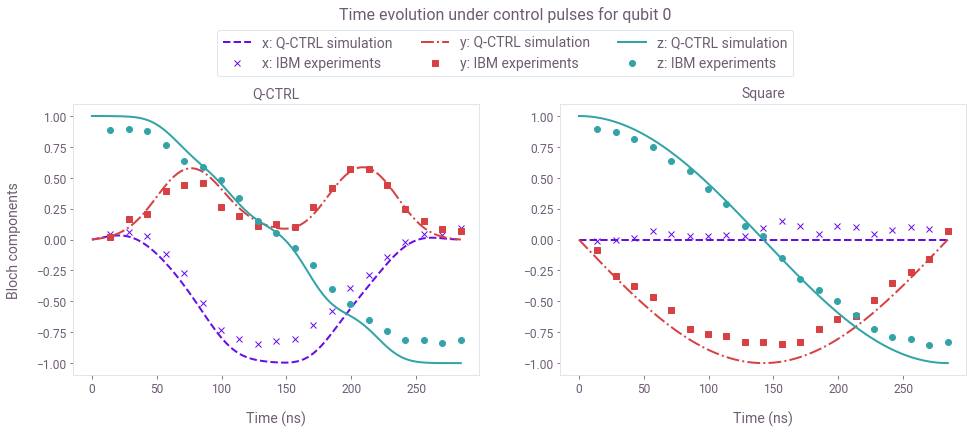Comparison between the Bloch vector components (x, y, z) for the IBM experiments (symbols) and numerical simulation (lines). The smooth and band-limited Q-CTRL pulse waveform successfully implements the desired gate on the IBM hardware.

Experimental robustness verification with a quasi-static scan

To demonstrate the pulse robustness, we perform the experimental detuning scan, similar to what was done for the theoretical controls. As an extra comparison case, added to the scan here is the default X-gate for this IBM backend. The steps to achieve this are combined in the next execution cell, as follows:

• Define detuning sweep interval,
• Create schedules and run jobs on IBM-Q,
• Extract results and produce robustness plot.
scheme_names.append("IBM default X-gate")
# Define detuning sweep interval
detuning_array = np.linspace(
qubit_frequency_updated - 1e6, qubit_frequency_updated + 1e6, 21
)
number_of_runs = 20
num_shots_per_point = 512

# Create schedules and run jobs
if use_IBM == True and use_saved_data == False:
drive_frequencies = [{drive_chan: freq} for freq in detuning_array]

pi_experiment_results = {}
for run in range(number_of_runs):
for scheme_name in scheme_names:
# Default IBM X-gate
if scheme_name == "IBM default X-gate":
pi_schedule = pulse.Schedule(name="IBM default X-gate")
pi_schedule |= inst_sched_map.get(
"u3", [qubit], P0=np.pi, P1=0.0, P2=np.pi
)
else:
pi_schedule = pulse.Schedule(name=scheme_name)
pi_schedule += Play(Waveform(waveform[scheme_name]), drive_chan)
pi_schedule += measure_schedule << pi_schedule.duration

pi_experiment_ibm = assemble(
pi_schedule,
backend=backend,
meas_level=2,
meas_return="single",
shots=num_shots_per_point,
schedule_los=drive_frequencies,
)

job = backend.run(pi_experiment_ibm)
print(job.job_id())
job_monitor(job)
pi_experiment_results[scheme_name + str(run)] = job.result(timeout=120)

# Extract results
detuning_sweep_results = {}
for scheme_name in scheme_names:
qctrl_sweep_values = np.zeros((number_of_runs, len(detuning_array)))
for run in range(number_of_runs):
i = 0
for result in pi_experiment_results[scheme_name + str(run)].results:
counts = result.data.counts["0x1"]
excited_pop = counts / num_shots_per_point
qctrl_sweep_values[run][i] = 1 - excited_pop
i = i + 1
detuning_sweep_results[scheme_name] = qctrl_sweep_values
filename = data_folder / ("detuning_sweep_results_" + timestr)
save_var(filename, detuning_sweep_results)

else:
filename = data_folder / "detuning_sweep_results_demo"
# Robustness plot
fig, ax = plt.subplots(figsize=(10, 5))
for scheme_name, probability in detuning_sweep_results.items():
ax.errorbar(
detuning_array / mega - qubit_frequency_updated / mega,
np.mean(probability, axis=0),
yerr=np.std(probability, axis=0)
/ np.sqrt(len(detuning_sweep_results[scheme_name])),
fmt="-o",
markersize=5,
capsize=3,
label=f"{scheme_name} run on IBM-Q",
color=colors[scheme_name],
)
if scheme_name != "IBM default X-gate":
plt.plot(
detuning_array / mega - qubit_frequency_updated / mega,
gate_infidelity["dephasing"][scheme_name] + 0.075,
linestyle="dashed",
label=f"{scheme_name} simulation",
color=colors[scheme_name],
)

fig.suptitle("Detuning scan")
plt.xlabel("Detuning (MHz)")
plt.ylabel("Ground state population")
plt.legend()
plt.show()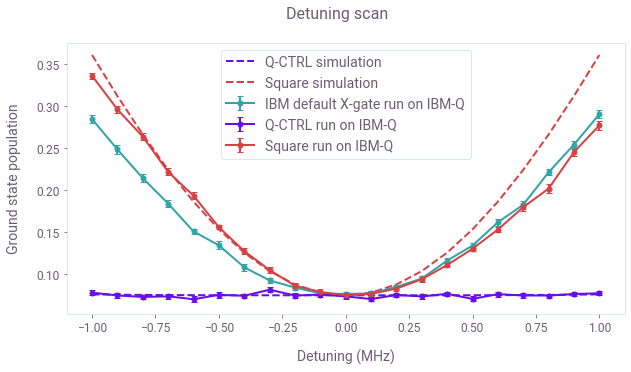Experimental (symbols) and simulated (lines) ground state populations as a function of the detuning. The Q-CTRL control solution is mostly flat across the detuning scan, showing its robustness against dephasing noise as compared to the primitive square pulse as well as the default IBM X-gate. The theoretical curves have been shifted up by $0.075$ to account for measurement errors in the experimental data.

Other noise sources: Q-CTRL pulse robustness against control error

Besides robustness to dephasing, we next use Boulder Opal to design solutions that are robust against errors in the control amplitudes. The workflow is exactly as in the dephasing case:

• Initialize Q-CTRL optimization parameters,
• Run optimization and extract results,
• Characterize robustness using filter functions,
• Calibrate and implement pulses,
• Verify robustness with an amplitude scan.

Creating pulses robust to control amplitude errors

The next cell contains all Q-CTRL commands to generate pulses for the control scenario defined previously. The only difference to the dephasing case is that the noise_operators are now time-varying terms of the control Hamiltonian.

number_of_segments = 256
number_of_optimization_variables = 64
segment_scale = 10
duration_int = number_of_segments * segment_scale
duration = duration_int * dt
cutoff_frequency = omega_max * 2.0
scheme_names = []

if use_saved_data == False:
robust_amplitude_controls = {}

graph = qctrl.create_graph()

# Create I & Q variables
I_values = graph.optimization_variable(
count=number_of_optimization_variables, lower_bound=-I_max, upper_bound=I_max
)
Q_values = graph.optimization_variable(
count=number_of_optimization_variables, lower_bound=-Q_max, upper_bound=Q_max
)

# Anchor ends to zero with amplitude rise/fall envelope
time_points = np.linspace(-1.0, 1.0, number_of_optimization_variables + 2)[1:-1]
envelope_function = 1.0 - np.abs(time_points)
I_values = I_values * envelope_function
Q_values = Q_values * envelope_function

# Create I & Q signals
I_signal = graph.pwc_signal(values=I_values, duration=duration)
Q_signal = graph.pwc_signal(values=Q_values, duration=duration)

# Apply the sinc filter
sinc_kernel = graph.sinc_convolution_kernel(cutoff_frequency)

I_signal_filtered = graph.convolve_pwc(pwc=I_signal, kernel=sinc_kernel)
Q_signal_filtered = graph.convolve_pwc(pwc=Q_signal, kernel=sinc_kernel)

# Re-discretize signal
I_signal = graph.discretize_stf(
stf=I_signal_filtered,
duration=duration,
segment_count=number_of_segments,
name="I",
)
Q_signal = graph.discretize_stf(
stf=Q_signal_filtered,
duration=duration,
segment_count=number_of_segments,
name="Q",
)

# Create Hamiltonian control terms
I_term = I_signal * sigma_x / 2.0
Q_term = Q_signal * sigma_y / 2.0

control_hamiltonian = I_term + Q_term

# Create dephasing noise term
amplitude = control_hamiltonian

# Create infidelity
infidelity = graph.infidelity_pwc(
hamiltonian=control_hamiltonian,
target=graph.target(X_gate),
noise_operators=[amplitude],
name="infidelity",
)

# Calculate optimization
result = qctrl.functions.calculate_optimization(
graph=graph, cost_node_name="infidelity", output_node_names=["I", "Q"]
)

print(f"Cost: {result.cost}")

robust_amplitude_controls["Q-CTRL"] = result.output

filename = data_folder / ("robust_amplitude_controls_" + timestr)
save_var(filename, robust_amplitude_controls)
else:
filename = data_folder / "robust_amplitude_controls_demo"
scheme_names.append("Q-CTRL")
# Create square pulse
square_pulse_durations = np.array([duration])
square_pulse_values_control = np.array([rabi_rotation / duration])
square_sequence = {
"I": [
{"duration": d, "value": v}
for d, v in zip(square_pulse_durations, square_pulse_values_control)
],
"Q": [
{"duration": d, "value": v}
for d, v in zip(square_pulse_durations, np.zeros([number_of_segments]))
],
}
scheme_names.append("Square")
# Combine all pulses into a single dictionary
gate_schemes = {**robust_amplitude_controls, **{"Square": square_sequence}}

scheme_names.append("IBM default X-gate")
for scheme_name, gate in gate_schemes.items():
fig = plt.figure()
fig.suptitle(scheme_name)
plot_controls(fig, gate)
plt.show()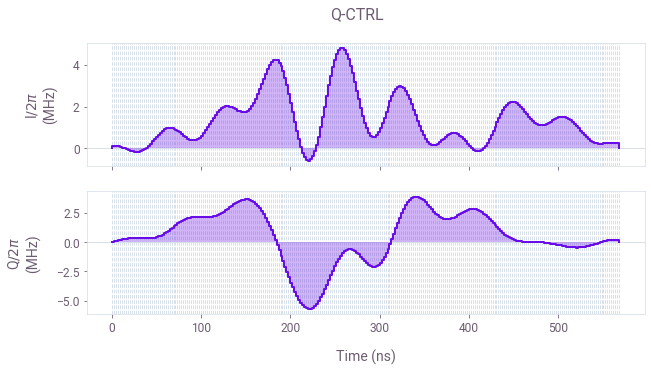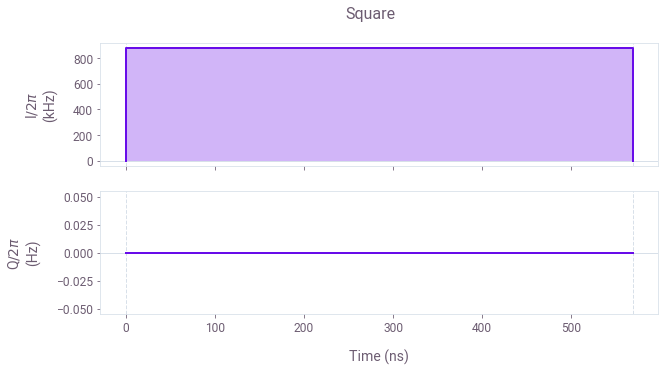$I(t)$ and $Q(t)$ components of the control pulses for the optimized (top) and primitive (bottom) controls. Note that the duration of the pulses has increased. This is to enable testing of the robustness of the pulses later, by scanning over a large range of control amplitude errors. Therefore using longer pulses (and lower Rabi rates) will help to always stay within the maximum Rabi rate bounds given by the backend.

Time evolution of the driven qubit: simulations and experiment

As for the amplitude robust pulses, we calibrate the control-error-robust pulses using the interpolation obtained previously and run them on the IBM machine to compare the theoretical and experimental time evolution of the qubit state under no added noise. The cell below combines the following steps:

• Pulse calibration,
• Set up IBM schedules,
• Run jobs on IBM-Q and extract results,
• Run Q-CTRL simulations,
• Plot results.
# Pulse calibration
waveform = {}
for scheme_name, control in gate_schemes.items():
I_values = [segment["value"] / 2 / np.pi for segment in control["I"]]
Q_values = [segment["value"] / 2 / np.pi for segment in control["Q"]]
if scheme_name == "Square":
A_I_values = np.repeat(
f_rabi_to_amp(I_values), segment_scale * number_of_segments
)
A_Q_values = np.repeat(
f_rabi_to_amp(Q_values), segment_scale * number_of_segments
)
else:
A_I_values = np.repeat(f_rabi_to_amp(I_values), segment_scale)
A_Q_values = np.repeat(f_rabi_to_amp(Q_values), segment_scale)
waveform[scheme_name] = A_I_values + 1j * A_Q_values

# Create IBM schedules
time_min = 64
time_max = duration_int
time_step = 128
num_shots_per_point = 2048
times_int = np.arange(time_min, time_max + time_step, time_step)
ibm_evolution_times = times_int * dt
num_time_points = len(times_int)

if use_IBM == True:
for scheme_name in gate_schemes.keys():
evolution_schedules = []
for meas_basis in bloch_basis:
for time_idx in times_int:
schedule = pulse.Schedule(
name="Basis_%s_duration_%d" % (meas_basis, time_idx)
)
schedule += Play(Waveform(waveform[scheme_name][:time_idx]), drive_chan)
if meas_basis == "x":
schedule += inst_sched_map.get("u2", , P0=0.0, P1=np.pi)
schedule += measure_schedule << schedule.duration
if meas_basis == "y":
schedule += inst_sched_map.get("u2", , P0=0.0, P1=np.pi / 2)
schedule += measure_schedule << schedule.duration
if meas_basis == "z":
schedule += measure_schedule << schedule.duration
evolution_schedules.append(schedule)

pulse_evolution_program[scheme_name] = assemble(
evolution_schedules,
backend=backend,
meas_level=2,
meas_return="single",
shots=num_shots_per_point,
schedule_los=[{drive_chan: qubit_frequency_updated}] * len(times_int) * 3,
)

if use_saved_data == False:
evolution_exp_results = {}
for scheme_name in gate_schemes.keys():
job = backend.run(pulse_evolution_program[scheme_name])
job_monitor(job)
evolution_exp_results[scheme_name] = job.result(timeout=120)

# Extract results
evolution_results_ibm = {}
for scheme_name in gate_schemes.keys():
evolution_basis = {}
for meas_basis in bloch_basis:
evolution_exp_data = np.zeros(len(times_int))
for idx, time_idx in enumerate(times_int):
counts = evolution_exp_results[scheme_name].get_counts(
"Basis_%s_duration_%d" % (meas_basis, time_idx)
)
excited_pop = 0
for bits, count in counts.items():
excited_pop += count if bits[::-1][qubit] == "1" else 0
evolution_exp_data[idx] = excited_pop / num_shots_per_point
evolution_basis[meas_basis] = evolution_exp_data
evolution_results_ibm[scheme_name] = evolution_basis

filename = data_folder / ("bloch_vectors_amplitude_" + timestr)
save_var(filename, evolution_results_ibm)
else:
filename = data_folder / "bloch_vectors_amplitude_demo"
else:
filename = data_folder / "bloch_vectors_amplitude_demo"
# Q-CTRL simulations
simulated_bloch = {}
gate_times = {}
for scheme_name, control in gate_schemes.items():
simulated_bloch[scheme_name], gate_times[scheme_name] = simulation_coherent(
control, 100
)

# Plot results
fig, axs = plt.subplots(1, len(gate_schemes.keys()), figsize=(15, 5))
fig.suptitle(f"Time evolution under control pulses for qubit {qubit}", y=1.15)

for idx, scheme_name in enumerate(gate_schemes.keys()):
ax = axs[idx]
for meas_basis in bloch_basis:
ax.plot(
gate_times[scheme_name] / nano,
simulated_bloch[scheme_name][meas_basis],
ls=bloch_lines[meas_basis],
color=bloch_colors[meas_basis],
label=f"{meas_basis}: Q-CTRL simulation",
)
ax.plot(
ibm_evolution_times / nano,
1 - 2 * evolution_results_ibm[scheme_name][meas_basis],
bloch_markers[meas_basis],
color=bloch_colors[meas_basis],
label=f"{meas_basis}: IBM experiments",
)

ax.set_title(scheme_name)
ax.set_xlabel("Time (ns)")

axs.set_ylabel("Bloch components")
hs, ls = axs.get_legend_handles_labels()
fig.legend(handles=hs, labels=ls, loc="center", bbox_to_anchor=(0.5, 1.02), ncol=3)

plt.show()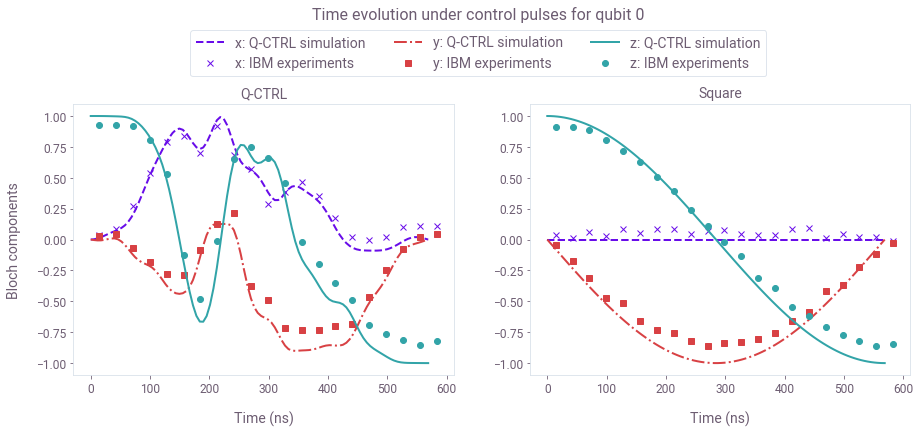Time evolution of the Bloch vector components (x, y, z) for simulations (lines) and experimental runs (symbols) under the control-robust pulses.

Experimental robustness verification with a quasi-static scan and filter functions

We again assess the sensitivity of the pulses to the noise using filter functions and quasi-static noise-susceptibility scans. The only difference here is the change in the noise process, which is achieved by replacing the dephasing term by noise on the controls. The filter functions calculated in the cell below use noise in the $I$ and $Q$ components of the controls.

sample_count = 4096
frequencies = np.logspace(-2, np.log10(omega_max), 2000)
filter_functions = {}

for scheme_name, control in gate_schemes.items():
durations = [segment["duration"] for segment in control["I"]]
I_values = np.array([segment["value"] for segment in control["I"]])
Q_values = np.array([segment["value"] for segment in control["Q"]])
phasors = I_values + Q_values * 1j

drive = qctrl.types.filter_function.Drive(
control=[
qctrl.types.ComplexSegmentInput(duration=duration, value=value)
for duration, value in zip(durations, phasors)
],
operator=sigma_p / 2,
noise=True,
)

filter_function = qctrl.functions.calculate_filter_function(
duration=np.sum(durations),
frequencies=frequencies,
sample_count=sample_count,
drives=[drive],
)

filter_functions[scheme_name] = filter_function.samples
Your task calculate_filter_function (action_id="619333") has started. You can use the qctrl.get_result method to retrieve previous results.

Your task calculate_filter_function (action_id="619335") has started. You can use the qctrl.get_result method to retrieve previous results.

We also demonstrate robustness by performing a quasi-static control-error scan, both numerically and experimentally. The steps follow the same structure as in the dephasing case and are combined in the next cell:

• Define control error range,
• Create schedules and run them on IBM-Q,
• Create Q-CTRL theoretical control error scan.
scheme_names = ["Q-CTRL", "Square", "IBM default X-gate"]
# Define control amplitude error range
amp_error = 0.25
amp_scalings = np.linspace(1 - amp_error, amp_error + 1, 21)

# Define schedules and run jobs
if use_IBM == True and use_saved_data == False:
IBM_default_X_gate_schedule = inst_sched_map.get(
"u3", [qubit], P0=np.pi, P1=0.0, P2=np.pi
)
pi_experiment_results = {}
for run in range(number_of_runs):
for scheme_name in scheme_names:
pi_schedule = []
for amp_scaling in amp_scalings:
if scheme_name == "IBM default X-gate":
this_schedule = pulse.Schedule(name=scheme_name)
for idx, inst in enumerate(
IBM_default_X_gate_schedule.instructions
):
if inst.name is None:
this_schedule |= pulse.Schedule(inst)
else:  # if "X90" in inst.name:
Ival = np.real(inst.instructions.pulse.samples)
Qval = np.imag(inst.instructions.pulse.samples)
phasors = Ival + 1j * Qval
this_schedule |= (
Play(
Waveform(phasors * amp_scaling), inst.channels
)
<< this_schedule.duration
)
this_schedule += measure_schedule << this_schedule.duration
pi_schedule.append(this_schedule)
else:
this_schedule = pulse.Schedule(name=scheme_name)
this_schedule += (
Play(Waveform(waveform[scheme_name] * amp_scaling), drive_chan)
<< 1
)
this_schedule += measure_schedule << duration_int
pi_schedule.append(this_schedule)

num_shots_per_point = 512

pi_experiment_ibm = assemble(
pi_schedule,
backend=backend,
meas_level=2,
meas_return="single",
shots=num_shots_per_point,
schedule_los=[{drive_chan: qubit_frequency_updated}]
* len(amp_scalings),
)
job = backend.run(pi_experiment_ibm)
print(job.job_id())
job_monitor(job)
pi_experiment_results[scheme_name + str(run)] = job.result(timeout=120)

# Extracting results
amplitude_sweep_results = {}
for scheme_name in scheme_names:
qctrl_sweep_values = np.zeros((number_of_runs, len(amp_scalings)))
for run in range(number_of_runs):
i = 0
for result in pi_experiment_results[scheme_name + str(run)].results:
counts = result.data.counts["0x1"]
excited_pop = counts / num_shots_per_point
qctrl_sweep_values[run][i] = 1 - excited_pop
i = i + 1
amplitude_sweep_results[scheme_name] = qctrl_sweep_values
filename = data_folder / ("amplitude_sweep_results_" + timestr)
save_var(filename, amplitude_sweep_results)
else:
filename = data_folder / "amplitude_sweep_results_demo"
# Q-CTRL control amplitude scan
gate_infidelity = {}
amplitude_array = np.linspace(-0.25, 0.25, 21)
gate_infidelity["amplitude"] = {
scheme_name: get_amplitude_scan(amplitude_array, control)
for scheme_name, control in gate_schemes.items()
}

Next, we plot the numerical filter functions and the control error scan.

fig, axs = plt.subplots(1, 2, figsize=(15, 5))
fig.suptitle("Robustness verification", y=1)

ax = axs
ax.set_xlabel("Frequency (Hz)")
ax.set_ylabel("Inverse power")
ax.set_title("Filter functions")
ax.set_xlim(np.min(frequencies), np.max(frequencies))

for scheme_name, _data in filter_functions.items():
inverse_powers = np.array([_d.inverse_power for _d in _data])
inverse_power_precisions = np.array([_d.inverse_power_uncertainty for _d in _data])

ax.loglog(
frequencies, inverse_powers, "-", label=scheme_name, color=colors[scheme_name]
)
y_upper = inverse_powers + inverse_power_precisions
y_lower = inverse_powers - inverse_power_precisions
ax.fill_between(
frequencies,
y_lower,
y_upper,
hatch="||",
alpha=0.35,
facecolor="none",
edgecolor=colors[scheme_name],
linewidth=0.0,
)
ax.legend()

ax = axs
ax.set_title("Robustness scan")
ax.set_ylabel("Ground state population")
ax.set_xlabel("Control amplitude error")
for scheme_name, probability in amplitude_sweep_results.items():
ax.errorbar(
amp_scalings - 1,
np.mean(probability, axis=0),
yerr=np.std(probability, axis=0)
/ np.sqrt(len(amplitude_sweep_results[scheme_name])),
fmt="-o",
capsize=5,
label=f"{scheme_name} run on IBM-Q",
color=colors[scheme_name],
)
if scheme_name != "IBM default X-gate":
ax.plot(
amp_scalings - 1,
gate_infidelity["amplitude"][scheme_name] + 0.071,
linestyle="dashed",
label=f"{scheme_name} simulation",
color=colors[scheme_name],
)
ax.legend()
plt.show()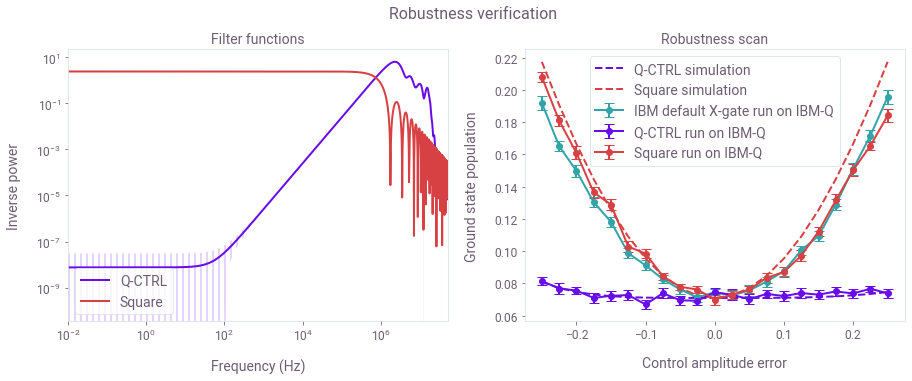Left plot: Filter functions for the control pulses under control noise in the $I$ component. Again, robustness is clearly demonstrated for the optimized Q-CTRL pulses. Right plot: Experimental (symbols) and simulated (lines) ground state populations as a function of the error in the control amplitude (note that the control amplitudes vary between -1 and 1). The Q-CTRL control solution is robust, with a flat response for a large range of control errors as compared to the primitive pulse. The theoretical curves have been shifted up by $0.071$ to account for measurement errors in the experimental data.